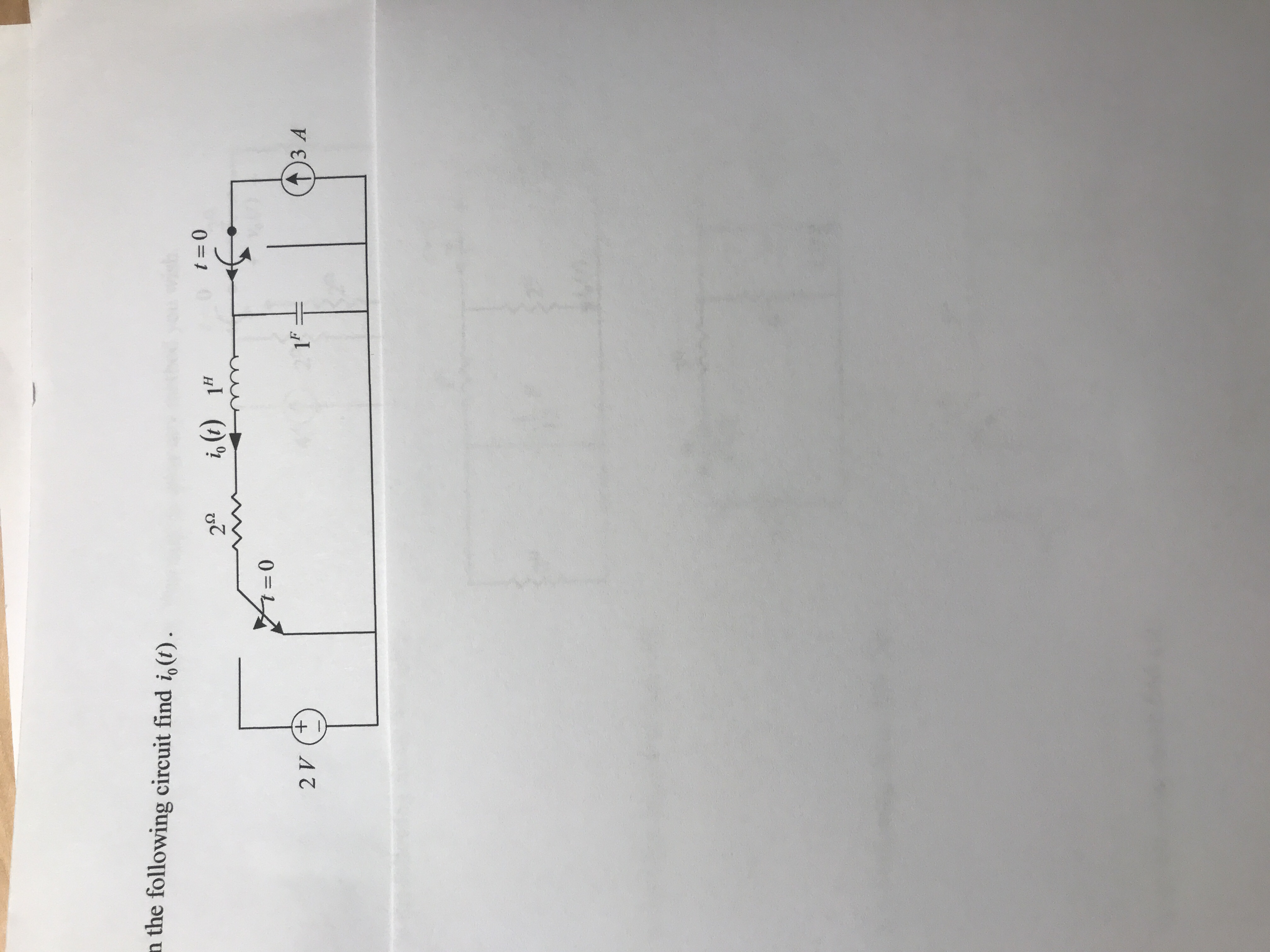a the following circuit find i,o1-0t=る(t)1н=0ЗА

Questionhelp_outlineImage Transcriptionclosea the following circuit find i,o 1-0 t= る(t) 1н =0 ЗА fullscreen
Step 1

We first find the initial and final values. Let us say that the voltage across the capacitor is v(t).

So, at time t = 0-, the circuit is at steady state :

Step 2

When t = 0+, the switch changed its position and the dc voltage source is connected to the circuit. By the continuity of capacitor voltage and inductor current, we know that:

Step 3

Transforming the circuit into L...

Want to see the full answer?

See Solution

Want to see this answer and more?

Our solutions are written by experts, many with advanced degrees, and available 24/7

See Solution
Tagged in

Electrical Engineering